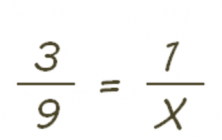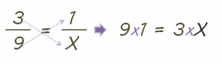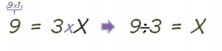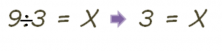Smartick is a fun way to learn math!Sep04

# Learn How to Solve Fractions with an Unknown Variable

In this post, we are going to learn to solve one of the situations in which we could encounter fractions with an unknown variable.

First, you should review what equivalent fractions are in some of our previous posts:

### We could encounter a mathematic expression with an unknown variable (x):As we already know, the “x” is the most common way of marking the unknown element.

Put in a real context it could be like this:

“Katrina is playing with her construction blocks and needs to make figures like these”“But Katrina does not have any more blocks of a one unit size.  She needs to make one more figure with the same design as the previous ones except with only one piece of a different color instead of three”

Visually her problem would be this:How can Katrina know how many blocks she should use?

As we know, in two equivalent fractions the cross-product of their elements is equivalent.To get our unknown (X) isolated on one of the equal sides, we should move all of its accompanying elements to the other equal side. In order to be able to make this change, the element that we move should invert its function (If you were adding switch to subtracting; if you were subtracting switch to adding; if you were dividing switch to multiplying.)

In this case, we move the 3 to the other equal side and since we were multiplying switch to dividing.Now we only have to carry out the operation. We divide 9 by 3:In this case, we already have solved Katrina’s problem.

Katrina should use 3 blocks to make her 1 piece.I hope from now on, thanks to this blog entry, you can easily understand and solve fraction problems with an unknown.

If you want to do more activities with fractions or unknowns, register with Smartick and discover a ton of things that you can learn in a variety of ways.